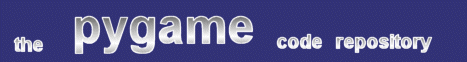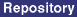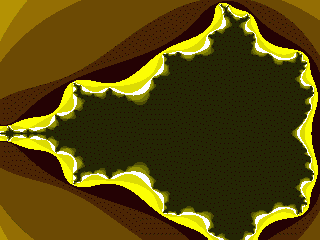### Title: Mandelbrot Animation Example

Author: Pete Shinners (pete at shinners.org)
Submission date: April 20, 2001

Description: Animates into a python generated mandelbrot imagepygame version required: Any with Numeric
SDL version required: Any
Python version required: >= 2.0

Comments: This code animates an exploration along the boundary of the Mandelbrot set. It requires Numeric, and accepts resolution and iterative depth parameters. Note that iterations greater than 9 will eventually produce an overflow error with the fast function; switch to the slow one for high depth values.

```
#!/usr/bin/env python

"""
Mandelbrot Animation
Pete Shinners
April 20, 2001

This is my attempt at doing a realtime python mandelbrot demonstation.
This mandelbrot algorithm orinally came from one of the Numeric python demos,
but I've gone and speeded it about about double. Still it's not what I'd
classify under "quick". The default settings of resolution 160x100 and
iterations of 6 runs reasonably quick on a Pentium2-300. increasing any of
these values looks really good, but slows things down a hunka lot!

Anyways, I'm don't entirely follow the mandelbrot algorithm myself. I'm
still not sure why this requires Complex number types, but my efforts to
replace with simple floats never worked. Owell, if anyone can speed this
up some, please share!

You may notice that while creating the mandelbrot it is created on one
axis, then transposed to be on the other. This was just a quicky addon
I did to make the mandelbrot appear in it's 'popular' orientation. You
could likely rework the algorithm a little so it just does it correctly,
but I don't imagine it would be any faster.

doh, i realized that in my "quicker" redo of this algorithm, the
complex numbers in the "Z" array will overflow with an iteration
value above 11. Too bad, cause it sure is pretty with that much detail.
Just to be fun then, I've included the unaltered mandelbrot function
which you can easily plugin and play with.
"""

from Numeric import *
import pygame, pygame.surfarray
from pygame.locals import *
from Numeric import *
import sys

def old_slow_mandelbrot(LowX, HighX, LowY, HighY, stepx, stepy, maxiter):
xx=arange(LowX,HighX,(HighX-LowX)/stepx)
yy=arange(HighY,LowY,(LowY-HighY)/stepy)*1j
c=ravel(xx+yy[:,NewAxis])
z=zeros(c.shape,Complex)
output = zeros(c.shape) + 1
for iter in range(maxiter):
z=z*z+c
finished=greater(abs(z),2.0)
c=where(finished,0+0j,c)
z=where(finished,0+0j,z)
output=where(finished,iter,output)
output.shape = (stepy, stepx)
return transpose(output)

def new_fast_mandelbrot(LowX, HighX, LowY, HighY, stepx, stepy, maxiter):
"creates a numeric array of the mandelbrot function"
if maxiter > 11: maxiter = 11  #for your own protection
xx = arange(LowX, HighX, (HighX-LowX)/stepx)
yy = arange(HighY, LowY, (LowY-HighY)/stepy) * 1.0j
#somtimes these arrays are too big by one element???
xx = xx[:stepx]
yy = yy[:stepy]
c = ravel(xx+yy[:,NewAxis])
z = zeros(c.shape, Complex)
output = zeros(c.shape) + 1
for iter in range(maxiter):
multiply(z, z, z)
add(output, greater(abs(z), 2.0), output)
output.shape = (stepy, stepx)
return transpose(output)

#you must choose wisely

#mandelbrot = old_slow_mandelbrot
mandelbrot = new_fast_mandelbrot

def main():
if len(sys.argv) != 4:
print 'Arguments    : xsize, ysize, mandel_iterations'
print 'Defaulting to: 160, 100, 6'
res = 160, 100
iterations = 6
else:
res = int(sys.argv), int(sys.argv)
iterations = int(sys.argv)
print 'Arguments    :', res, iterations

pygame.init()
screen = pygame.display.set_mode(res, 0, 8)

l_val, r_val = -2.1, 0.7
t_val, b_val = -1.2, 1.2

l_inc, r_inc = .03, -.02
t_inc, b_inc = .03, -.005
while 1:
if pygame.event.peek([QUIT,KEYDOWN]): break

mand = mandelbrot(l_val, r_val, t_val, b_val, res, res, iterations)
multiply(mand, 36, mand) #this just chooses 'prettier' color index values
pygame.surfarray.blit_array(screen, mand)
pygame.display.flip()

l_val += l_inc
r_val += r_inc
t_val += t_inc
b_val += b_inc

if __name__ == '__main__': main()

```

 Feedback